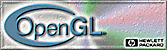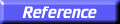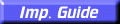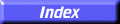# gluSphere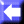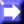gluSphere: draw a sphere.

##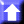C Specification

```void gluSphere(
GLint	 slices,
GLint	 stacks)
```

##Parameters

Specifies the radius of the sphere.
slices
Specifies the number of subdivisions around the Z axis (similar to lines of longitude).
stacks
Specifies the number of subdivisions along the Z axis (similar to lines of latitude).

##Description

gluSphere draws a sphere of the given radius centered around the origin. The sphere is subdivided around the Z axis into slices and along the Z axis into stacks (similar to lines of longitude and latitude).

If the orientation is set to GLU_OUTSIDE (with gluQuadricOrientation), then any normals generated point away from the center of the sphere. Otherwise, they point toward the center of the sphere.

If texturing is turned on (with gluQuadricTexture), then texture coordinates are generated so that t ranges from 0.0 at Z =
radius to 1.0 at Z = radius (t increases linearly along longitudinal lines), and s ranges from 0.0 at the +Y axis, to 0.25 at the +X axis, to 0.5 at theY axis, to 0.75 at theX axis, and back to 1.0 at the +Y axis.

gluCylinder,
gluDisk,